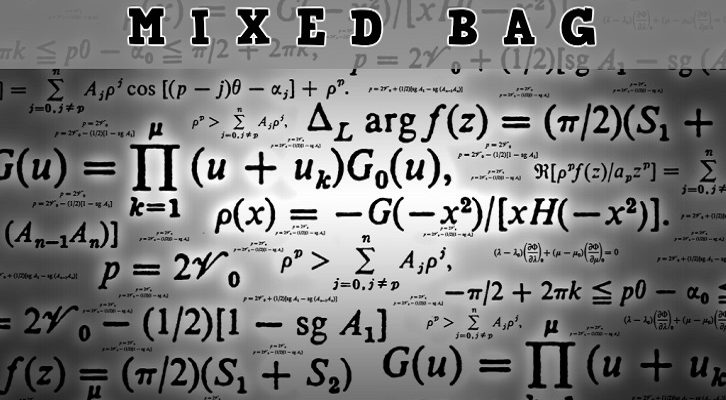# CAT Data Sufficiency Solutions

Have given below solutions to the questions on data sufficiency

1. A point P is identified as P(m,n). What is the ratio AP:BP given that the points A and B are identified as A(5,-4) and B(1,6)?

Stmt 1. m = 3. Not sufficient.
Stmt 2. n = 2.5 Not sufficient.

Either statement alone is not sufficient. Both put together, we can answer the questions. Ans B

2. A point P is identified as P(m,n). What is the ratio AP:BP given that the points A and B are identified as A(5,-4) and B(5,6)?

Stmt 1. m = 5. Not sufficient. It tells us that all points lie on the line x = 5, but this is not enough
Stmt 2. n = 3 Not sufficient.

Both put together, this is enough.

In the above question, would n = 1 have been sufficient? Think about that.

3. A survey of 100 people tried to find the number of people who can write with both their left and right hands. What is the maximum number of people who could write left-handed and right-handed?

Stmt 1. 50 people can write only with their left hand. 40 people can write only with their right hand. Sufficient: Maximum of 10 people could write left-handed and right-handed.
Stmt 2. 50 people can write with their left hand. 40 people can write with their right hand. Sufficient: Maximum of 40 people could write left-handed and right-handed.

4. What is the slope of a line?

Stmt 1: The line makes 135 degrees with the negative direction of x – axis. Sufficient: The line makes 45 degrees with positive x axis. This should be enough.
Stmt 2: The line makes an isosceles right triangle with the coodinate axes and the product of the intercepts is negative. Sufficient: Either both intercepts are positive and equal or negative and equal. Slope = -1

### Related posts

1.TechShady says:
2.Vicky Sadhu says: